December 7, 2021

# Class 10 Maths Chapter 9 Some Applications Of Trigonometry

In chapter 8, we were introduced to the concept of trigonometry. We learned trigonometric ratios, values of some specific angles, the inverse of those ratios, and some identities. In class 10 maths chapter 9 some applications of trigonometry as the name suggest, we will learn how trigonometry can be implemented in real life. In other words, we will learn the real-life applications of trigonometry in this chapter.

We will apply all that we have learned in the previous chapter to solve the problems that will be presented in this chapter. You will get a good idea of how height and distance can be calculated based on just the trigonometric ratios.

Trigonometry is one of the oldest branches of maths, and it is being used from ancient times in different walks of life like astronomy, geography, constructing maps, navigation, etc. In this chapter, we will see some basic applications of this branch in daily life hence setting a base for higher classes.

## Class 10 Maths Chapter 9 Some Applications Of Trigonometry

The application of trigonometry is one of the most important chapters from the exam perspective as high marking questions are asked from this chapter. This is why it is absolutely necessary that you understand every bit of it, and as we know, without having the basics clear, one can not get in-depth of any concept. So, let’s first take a look at all the important formulas that we will need in class 10 maths chapter 9 some applications of trigonometry.

Trigonometric ratios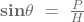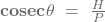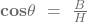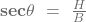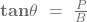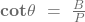Values of some specific angles
∠A 30º 45º 90º
sin A 0 ½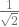1
cos A 1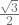0
tan A 0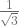1 not defined
cosec A not defined 2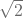1
sec A 1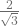not defined
cot A not defined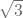1 0
complementary angles
sin (90°– A) = cos A cos (90°– A) = sin A
tan (90°– A) = cot A cot (90°– A) = tan A
sec (90°– A) = cosec A cosec (90°– A) = sec A
Trigonometric identities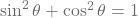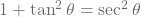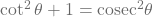The above-given tables are basically the summary of all that we have read in the previous chapter. These tables will help you recoil and memorize all the formulas so that you can practice class 10 maths chapter 9 some applications of trigonometry in a better way.

### Class 10 Maths Chapter 9 Some Applications Of Trigonometry

Since now we have the much-needed formula cheat sheet for this chapter, we can proceed to learn the new things that will come in chapter 9. First of all, we will know about all the important terms that will be there in this chapter so that there is no hindrance when we will get into the details of this chapter.

What is ‘line of sight’?

Imagine that you are standing on the ground and looking at top of a tower. Now, if someone were to draw a line from your eyes to the top of that tower, then that line will be called the line of sight. In this case, you are the observer and the top of the tower is the point that you are observing. So, the line of sight is an imaginary line that is drawn from the eyes of an observer to the point that is being viewed by the observer.

As we read in the previous chapter that trigonometry is all about triangles, so, the line of sight usually makes the hypotenuse of the triangle.

What is the horizontal level?

If we draw a straight imaginary line from your eyes to the point above which the object (that you are observing) is located, then it will be called the horizontal or horizontal level. The horizontal level generally forms the base for the triangle.

What is the angle of elevation?

The angle between the line of sight and the horizontal level is called the angle of elevation (assuming that the point of viewing is above the horizontal level).

What is the angle of depression?

Now let’s imagine that you are standing on top of that tower (such that your eyes in level with the top of the tower) and looking at the point where you were standing before. In such a case, the line drawn from your eyes to that point on the ground will be the line of sight, the horizontal line will be the straight line from your eyes level, and the angle between the line of sight and horizontal will be the angle of depression.

For the angle of elevation, the point which is being observed is above the horizontal level, while for the angle of depression that point is below the horizontal level.

#### Class 10 Maths Chapter 9 Some Applications Of Trigonometry

So far one thing must have been clear by now that the height or length of an object or the distance between two distant objects can be determined with the help of trigonometric ratios. On the NCERT book it looks like a very small chapter with just 1 exercise, but don’t misunderstand it to be a small one.

There are tones of questions that may come from this chapter, so it’s recommended to consider practicing from refreshers as well but only after completing the NCERT solutions first. Do practice as much as you can because it more is less when it comes to class 10 maths chapter 9 some applications of trigonometry. Here is a blueprint for this chapter.

Class 10 maths chapter 9 some applications of trigonometry
3 Marker 1 question
5 Marker 1 question
Total questions 2
Total marks 8

Since the above-given blueprint is made on the marking scheme as released by CBSE on their official website for this year, therefore, it is subject to change. The number of questions and the marks related to them may be different in the exam, therefore students are advised to be prepared for all kinds of situations.

As we can see in the table, for this year, a total of 2 questions will be asked from this chapter for a total of 8 marks. Out of these 2 questions, one question will be of 3 marks, while the other one will be of 5 marks. Since both the questions are high markers, therefore, it can be said that the level of the questions will be a bit difficult, so be prepared for it.